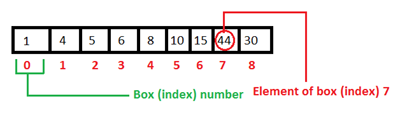# A little about array in Java

I have seen many of my friends to have trouble with the problems in array. So, this time I have selected to write with array…don’t know how much effective it would be to you guys…

First of all, what is array? Normally we can say that array is a static memory allocation. For example we can think an array as a number of conjoined boxes where we can store our desired values of same data type. Now the question may arise that we can use normal variables to store data, why use array? The answer is we should use array because it saves our time and minimizes the memory usage of the machine. For example: suppose that you have to store marks of 5000 students of your university. Now if you want to store those marks using normal variables firstly you have to select 5000 variables (which would be horrible!). Then, you will need to declare each variable (int a, b, c…up to 5000 variables). Than you have to initialize each variable too, haven’t you? In this case if you use array your task will become easier. All you have to do is, firstly you have to declare an array. Secondly, you have to initialize that the array will be consist of 5000 indexes it means the array will consist of 5000 conjoined boxes where the marks will be stored.How to declare an array?

Declaration of arrays is nearly same as the declaration of normal variables. We have to put [] between the data type and the array name.

Example:

```int [] MyFirstArray;
```

How to initialize the dimension of an array?

An array can be single or multi-dimensional. After declaring the array you need to set the dimension of the array. You can do it by this way.

```MyFirstArray [] = new int ;
```

It means that the array named MyFirstArray will have 10 boxes to store your value. Note that, the box number counts from 0, so the box number will be from 0 to 9.

How to initialize the value of each index (box)?

Now it’s needed to initialize the values of each index (box or subscript).  You can do it in two ways (as far I know). The first way is to initialize values of index individually.

Example:

```MyFirstArray  = 5;
MyFirstArray = 3;
```

Like this up to MyFirstArray.

The second way is, you can initialize all the values in one statement (This is a positive point of array).

Example:

```MyFirstArray[] = {1, 4, 5, 6, 5, 6,};
```

Note that: In this way if you do not initialize all index of the array rest of the index’s (box’s) element will set to zero by default. For example: if you set your array’s dimension to 10 and initialize 5 out of them using the second method rest of the index’s (box’s) value will set to zero.

I will post about two dimensional array on my next post… 🙂

Happy programming